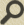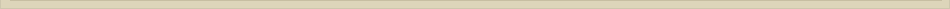# Lesson 5: Open Sentences and Quantifiers

All course documents available here.

# Problems

1.3.1 Translate the following English sentences into symbolic sentences with quan­tifiers. The universe for each is given in parentheses.

(a) Not all precious stones are beautiful. (All stones)
(b) All precious stones are not beautiful. (All stones)
(c) Some isosceles triangle is a right triangle. (All triangles)
(d) No right triangle is isosceles. (All triangles)
(e) All people are honest or no one is honest. (All people)
(f) Some people are honest and some people are not honest. (All people)
(g) There is a smallest positive integer. (Real numbers)
(h) Between any real number and any larger real number, there is a rational number. (Real numbers)
(i) No one loves everybody. (All people)
(j) Everybody loves someone. (All people)
(k) For every positive real number x, there is a unique real number y suchthat 2 = x. (Real numbers)
(I) For every complex number, there is at least one complex number such that the product of the two complex numbers is a real number. (Real numbers, as the coefficients)
(m) For every nonzero complex number, there is a unique complex number such that their product is π. (Real numbers, as the coefficients)

1.3.2 For each of the propositions in exercise I, write a useful denial, and give a translation into ordinary English. (Solution not available yet; Solve it!)

1.3.3 Give two proofs of Theorem 1.3(b). (Solution not available yet; Solve it!)

Theorem 1.3(b): If A (x) is an open sentence with variable x, then ¬(∃x)A(x) is equivalent to (∀x)¬A(x).

1.3.4 Which of the following are true? The universe for each is given in parentheses.  (Solution not available yet; Solve it!)

(a) (∀x)(x + x ≥ x) (Real numbers)
(b) (∀x)(x + x ≥ x) (Natural numbers)
(c) (∃x)(2x + 3 = 6x + 7) (Natural numbers)
(d) (∃x)(3^x = x^2 ) (Real numbers)
(e) (∃x)(3^x = x) (Real numbers)
(f) (∃x)(3(2 – x) = 5 + 8(1 – x)) (Real numbers)
(g) (∀x)(x^2 + 6x + 5 ≥ 0) (Real numbers)
(h) (∀x)(x^2 + 4x +5 ≥ 0) (Real numbers)
(i) (∃x)(x^2 + x + 41 is prime) (Natural numbers)
(j) (∀x)(x^2 + x + 41 is prime) (Natural numbers)
(k) (∀x)(x^3 + 17x^2 + 6x + 100 ≥ 0) (Real numbers)

1.3.5 Give an English translation for each. The universe is given in parentheses.  (Solution not available yet; Solve it!)

(a) (∀x)(x ≥ I) (Natural numbers)
(b) (∃!x)(x ≥ 0 ∧ x ≤ 0) (Real numbers)
(c) (∀x)( x is prime ∧ x ≠ 2 ⇒ x is odd) (Natural numbers)
(d) (∃!x)(ln(x) = 1) (Real numbers)
(e) ¬(∃x)(x^2 < 0 ) (Real numbers)
(f) (∃!x)(x^2 = 0) (Real numbers)
(g) (∀x)(x is odd ⇒ x^2 is odd) (Natural numbers)

1.3.6 Which of the following are true for the universe of all real numbers? (Solution not available yet; Solve it!)

(a) (∀x)(∃y)(x + y = 0).
(b) (∃x)(∀y)(x + y = 0).
(c) (∃x)(∃y)(x^2 + y^2 = -1)
(d) (∀x)[x > 0 ⇒ (∃y)(y < 0 ∧ xy > 0)]
(e) (∀y)(∃x)(∀z)(xy = xz)
(f) (∃x)(∀y)(x ≤ y)
(g) (∀y)(∃x)(x ≤ y)
(h) (∃!y)(y < 0 ∧ y + 3 > 0)
(i) (∃!x)(∀y)(x = y^2)
(j) (∀y)(∃!x)(x = y^2)
(k) (∃!x)(∃!y)(∀w)(w^2 > x – y)

1.3.7 (a) Give a proof of (∃!x)P(x) ⇒ (∃x)P(x). (b) Show that the converse of the conditional sentence in (a) is false. (Solution not available yet; Solve it!)

1.3.8 Write a symbolic translation of the Mean Value Theorem from calculus. (Solution not available yet; Solve it!)

1.3.9 Write a symbolic translation of the definition of limf(x) = L, x->a. Find a useful denial and give an idiomatic English version. (Solution not available yet; Solve it!)

1.3.10 Which of the following are denials of (∃!x)P(x)? (Solution not available yet; Solve it!)
(a) (∀x)(P(x))∨(∀x)(¬P(x))
(b) (∀x)(¬P(x))∨(∃y)(∃z)(y ≠ z ∧ P(y) ∧ P(z))
(c) (∀x)[P(x) ⇒ (∃y)(P(y) ∧ x ≠ y)]
(d) ¬(∀x)(∀y)[(P(x) ∧ P(y)) ⇒ x = y]

1.3.12 Riddle: What is the English translation of the symbolic statement ∀∃∃∀? (Solution not available yet; Solve it!)